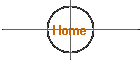How Far/How Faint

How much brighter is the Sun as viewed from the planet Mercury as compared to Earth? How much fainter is it at Neptune?  How strong is the Sun's gravitational pull on the Voyager 1 spacecraft now as compared to when it was at Jupiter?  How much pull does the Sun exert on the nearest star?

Actually, all of these questions can be answered through a very simple mathematical relationship known as the inverse square law.

It is a simple division problem that can be applied to a variety of interesting situations that affect planets, spacecraft that venture out into deep space and a number of other natural phenomena.

The equation relates the relative distances of two objects as compared to a third. Typically one of the objects is Earth, the second is a spacecraft and the third is the Sun. To begin, lets make some generalizations. There is a certain amount of sunlight reaching Earth at any given moment. This is not an absolute quantity since Earth is closer to the Sun at some times of the year verses others and the number of sunspots effects the Sun's energy output, but overall the Sun is remarkably constant in its behavior. If it were not, life on Earth might be impossible.

We can describe the amount of the Sun's energy reaching Earth as 1 solar constant. The average distance from the Sun to Earth is 149,597,870.66 kilometers (92,955,807.25 miles) which we can simplify to what astronomers call 1 Astronomical Unit or 1 AU.   So Earth is 1 AU from the Sun and receives 1 solar constant.   This will help keep the math easy.

The relationship can be expressed most simply as: 1/d2 (one over the distance squared) where d = distance as compared to Earth's distance from the Sun (for our first examples).

Let's start with sunlight as an example. At 1 AU, Earth receives 1 unit of sunlight; what we generally might associate with a bright sunny day at noon. How much sunlight would a spacecraft receive if it were twice as far from the Sun as Earth? Your first guess might be that, since it is twice as far it will only receive half as much (not twice as much since it is farther away).

The distance from the Sun to the spacecraft would be 2 AUs so... d = 2.  If we plug that into the equation 1/d2 = 1/22 = 1/4 = 25% The spacecraft is getting only one quarter of the amount of sunlight that would reach it if it were near Earth.  This is because the light is being radiated from the Sun in a sphere.  As the distance from the Sun increases the surface area of the sphere grows by the square of the distance.  That means that there is only 1/d2 energy falling on any similar area on the expanding sphere.

Now lets try it for another real place. Mars is at a distance of 1.5 AUs from the Sun. 1/d2 = 1/1.52 = 1/2.25 = 44%.  There is less than half as much sunlight falling on the surface of Mars as on Earth!  Jupiter is at 5.2 AUs so 1/d2 = 1/5.22 = 1/27 = 3.7%.  Neptune is at 30 AUs so 1/d2 = 1/302 = 1/900 or  0.1% !  Noon on Neptune is like very deep twilight on Earth!

What happens as we approach the Sun? Common sense tells us that the Sun will be brighter and the inverse square law tells us how much brighter. Mercury is at 0.387 AUs. 1/d2 = 1/0.3872 =  1/.15 = 666.67%, almost seven times brighter! We can use this method to compare any spot in the Universe if we describe its distance as compared to Earth relative to the Sun.

 Planet Dist. (in AUs) Mercury 0.387 Venus 0.723 Earth 1.000 Mars 1.523 Jupiter 5.202 Saturn 9.538 Uranus 19.181 Neptune 30.057 Dwarf Planet Pluto (min.)* 29.69 Pluto (avg.) 39.44 Pluto (max.) 49.19 a Centauri** 272,000

* Pluto's eccentric orbit carries it closer to the Sun than Neptune occasionally.

** "Alpha" Centauri - the star system (three stars) nearest our Sun; approximately 4.3 light years away (63,240 AU/light year).   Not visible from U.S. except in Hawaii.

We have been comparing sunlight but this is exactly the same method that we would use for any other form of randomly radiated energy such as heat, ultraviolet or x-rays, magnetic field strength or gravity. The gravitational tug that the Sun exerts on Earth can be compared to the Sun's tug on Mercury, Pluto, spacecraft or the stars.

Source:  Educator's Guide to The Inverse Square Law
Jet Propulsion Laboratory Public Education Office, Mail Stop CS-530, 4800 Oak Grove Drive, Pasadena CA 91109.  Educators can write to the JPL Teaching Resource Center, at the address above, for additional materials.

11/2011

 10/01/2017